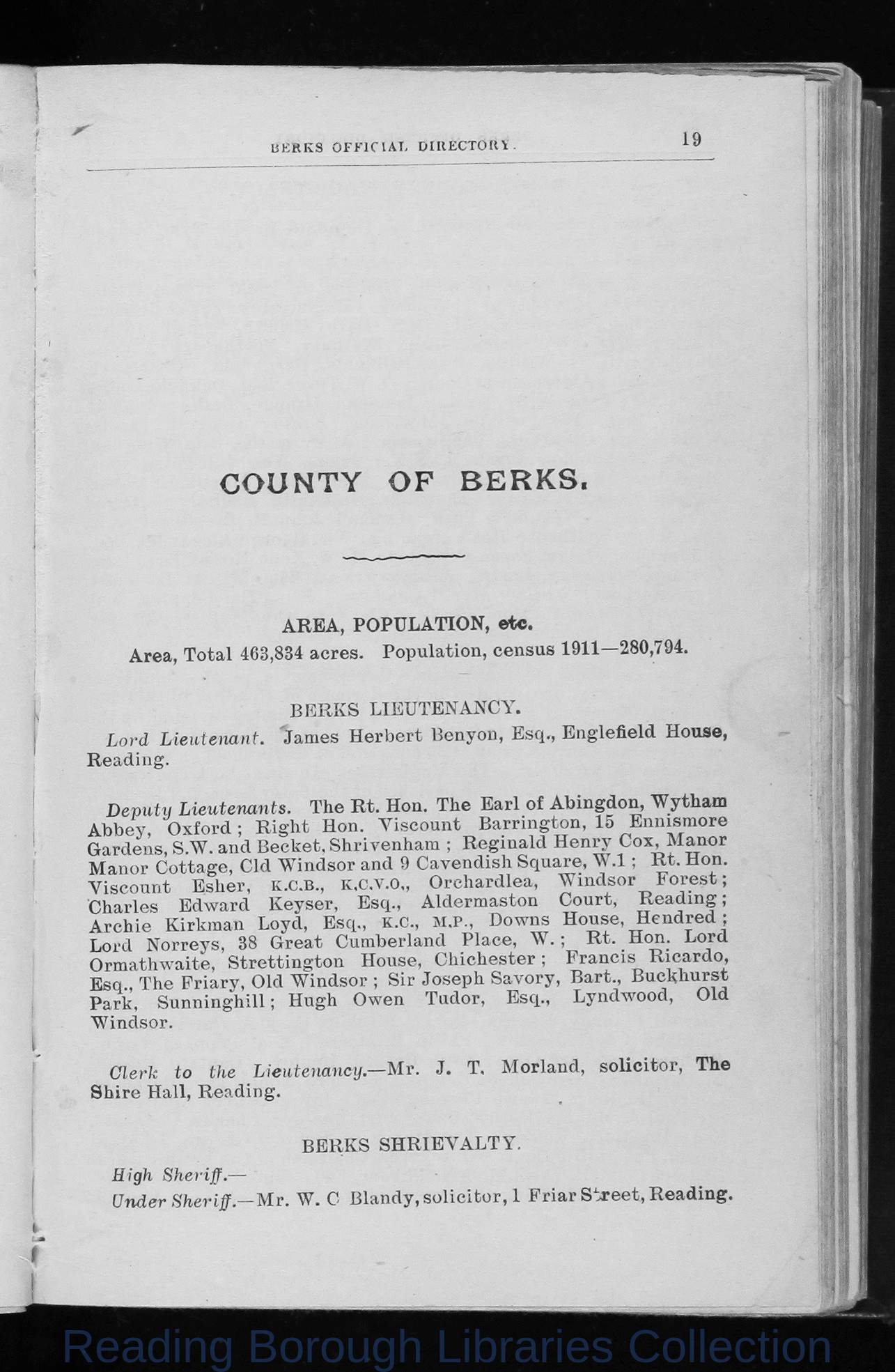## Image Details

There is no information available.

## OCR Text

19

BERKS OFFICIAI, DIRECTORY

COUNTY

OF

BERKS.

AREA, POPULATION, etc.
A r e a , T o t a l 4 6 3 ,8 3 4 a c r e s .

P o p u l a t i o n , c e n s u s 1 9 1 1 â 2 8 0 ,7 9 4 .

B E R K S

Lord L ieutenant.

Jam es

L IE U T E N A N C Y .

H erb e rt

B e n y o n , E s q ., E n g l e f ie l d

House,

R e a d in g .

D ep u ty Lieutenants.
A b b e y

O x fo rd

;

T h e R t. H on. T h e

R ig h t

H on.

G a r d e n s , S .W . a n d B e c k e t . S h r i v e n h a m
M a n o r C o tta g e , C ld
Y is c o u n t
C h a r le s
A rc h ie
L ord

E sh er,
E d w a rd

E sq

N o rreys,
, T h e

P ark ,

Loyd ,

38

G rea t

S u n n in g h ill;

R e g in a ld

K .C .,

O r c h a r d le a ,

15

M .P .,

H ouse,

W

.l:

W in d s o r
C o u rt,

D ow n s

P la c e ,

Wytham

E n n is m o r e

H en ry C o x , M an or

A ld e r m a s to n

C u m b e r la n d

S tre ttin g to n

F r i a r y , O ld

E s q .,

E s q .,

;

B a rr in g to n ,

9 C a v e n d is h S q u a r e , W

K .c .v .o ,,

K ey se r,

K ir k m a n

O r m a th w a ite ,

W in d s o r a n d
K .C .B .,

E a rl o f A b in g d o n ,

Y is c o u n t

.;

R t.

F o re st;
R e a d in g ;

H ou se,

C h ic h e s te r ;

R t. H on.

H e n d re d ;
H on.

F r a n c is
B a r t.,

H ugh

L yn d w o o d ,

O w en

T u d o r,

E s q .,

L ord

R ic a r d o ,

W in d s o r ; S ir J o s e p h S a v o r y ,

B u ck h u rst
O ld

W in d s o r .

Clerk to

the

Lieutenancy. â

M r.

J.

T,

M o r la n d ,

solicitor, The

S h i r e H a ll, R e a d in g .

B E R K S

High Sheriff . â
Under S h eriffâ M r.

W . C

S H R IE V A L T Y .

B la n d y , s o lic ito r , 1 F r ia r S t r e e t, R e a d in g .